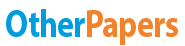# Introduction to Hypothesis Testing

Essay by   •  March 5, 2011  •  Essay  •  261 Words (2 Pages)  •  2,272 Views

## Essay Preview: Introduction to Hypothesis Testing

Report this essay
Page 1 of 2

6: Introduction to Hypothesis Testing

Significance testing is used to help make a judgment about a claim by addressing the question, Can the observed difference be attributed to chance? We break up significance testing into three (or four) steps:

Step A: Null and alternative hypotheses

The first step of hypothesis testing is to convert the research question into null and alterative hypotheses. We start with the null hypothesis (H0). The null hypothesis is a claim of "no difference." The opposing hypothesis is the alternative hypothesis (H1). The alternative hypothesis is a claim of "a difference in the population," and is the hypothesis the researcher often hopes to bolster. It is important to keep in mind that the null and alternative hypotheses reference population values, and not observed statistics.

Step B: Test statistic

We calculate a test statistic from the data. There are different types of test statistics. This chapter introduces the one-sample z-statistics. The z statistic will compare the observed sample mean to an expected population mean μ0. Large test statistics indicate data are far from expected, providing evidence against the null hypothesis and in favor of the alternative hypothesis.

Step C: p Value and conclusion

The test statistic is converted to a conditional probability called a P-value. The P- value answers the question "If the null hypothesis were true, what is the probability of observing the current data or data that is more extreme?"

Small p values provide evidence against the null hypothesis because they say the observed data are unlikely when the null hypothesis is true. We apply the following conventions:

o When p value > .10

...

...